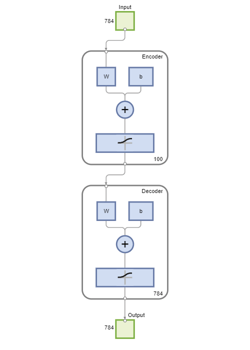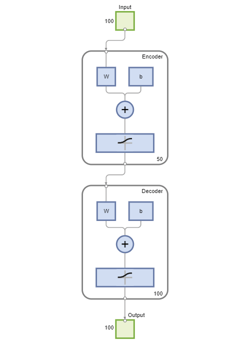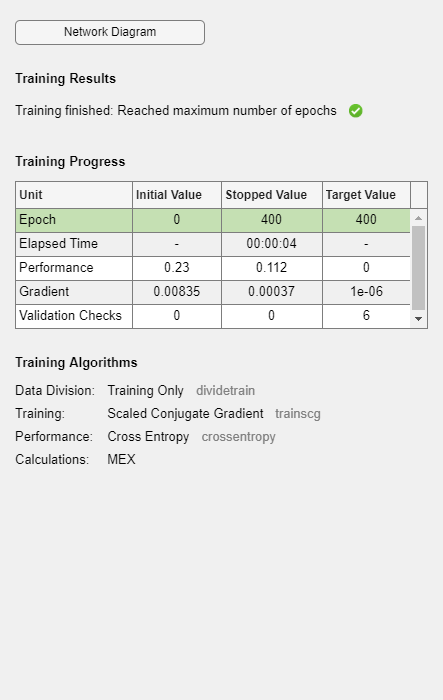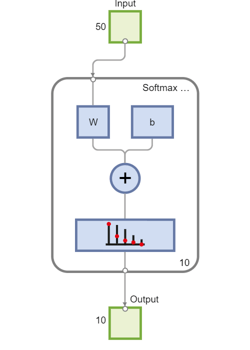Documentation

## Train Stacked Autoencoders for Image Classification

This example shows how to train stacked autoencoders to classify images of digits.

Neural networks with multiple hidden layers can be useful for solving classification problems with complex data, such as images. Each layer can learn features at a different level of abstraction. However, training neural networks with multiple hidden layers can be difficult in practice.

One way to effectively train a neural network with multiple layers is by training one layer at a time. You can achieve this by training a special type of network known as an autoencoder for each desired hidden layer.

This example shows you how to train a neural network with two hidden layers to classify digits in images. First you train the hidden layers individually in an unsupervised fashion using autoencoders. Then you train a final softmax layer, and join the layers together to form a stacked network, which you train one final time in a supervised fashion.

### Data set

This example uses synthetic data throughout, for training and testing. The synthetic images have been generated by applying random affine transformations to digit images created using different fonts.

Each digit image is 28-by-28 pixels, and there are 5,000 training examples. You can load the training data, and view some of the images.

```% Load the training data into memory [xTrainImages,tTrain] = digitTrainCellArrayData; % Display some of the training images clf for i = 1:20 subplot(4,5,i); imshow(xTrainImages{i}); end ```The labels for the images are stored in a 10-by-5000 matrix, where in every column a single element will be 1 to indicate the class that the digit belongs to, and all other elements in the column will be 0. It should be noted that if the tenth element is 1, then the digit image is a zero.

### Training the first autoencoder

Begin by training a sparse autoencoder on the training data without using the labels.

An autoencoder is a neural network which attempts to replicate its input at its output. Thus, the size of its input will be the same as the size of its output. When the number of neurons in the hidden layer is less than the size of the input, the autoencoder learns a compressed representation of the input.

Neural networks have weights randomly initialized before training. Therefore the results from training are different each time. To avoid this behavior, explicitly set the random number generator seed.

```rng('default') ```

Set the size of the hidden layer for the autoencoder. For the autoencoder that you are going to train, it is a good idea to make this smaller than the input size.

```hiddenSize1 = 100; ```

The type of autoencoder that you will train is a sparse autoencoder. This autoencoder uses regularizers to learn a sparse representation in the first layer. You can control the influence of these regularizers by setting various parameters:

• `L2WeightRegularization` controls the impact of an L2 regularizer for the weights of the network (and not the biases). This should typically be quite small.

• `SparsityRegularization` controls the impact of a sparsity regularizer, which attempts to enforce a constraint on the sparsity of the output from the hidden layer. Note that this is different from applying a sparsity regularizer to the weights.

• `SparsityProportion` is a parameter of the sparsity regularizer. It controls the sparsity of the output from the hidden layer. A low value for `SparsityProportion` usually leads to each neuron in the hidden layer "specializing" by only giving a high output for a small number of training examples. For example, if `SparsityProportion` is set to 0.1, this is equivalent to saying that each neuron in the hidden layer should have an average output of 0.1 over the training examples. This value must be between 0 and 1. The ideal value varies depending on the nature of the problem.

Now train the autoencoder, specifying the values for the regularizers that are described above.

```autoenc1 = trainAutoencoder(xTrainImages,hiddenSize1, ... 'MaxEpochs',400, ... 'L2WeightRegularization',0.004, ... 'SparsityRegularization',4, ... 'SparsityProportion',0.15, ... 'ScaleData', false); ```

You can view a diagram of the autoencoder. The autoencoder is comprised of an encoder followed by a decoder. The encoder maps an input to a hidden representation, and the decoder attempts to reverse this mapping to reconstruct the original input.

```view(autoenc1) ```### Visualizing the weights of the first autoencoder

The mapping learned by the encoder part of an autoencoder can be useful for extracting features from data. Each neuron in the encoder has a vector of weights associated with it which will be tuned to respond to a particular visual feature. You can view a representation of these features.

```figure() plotWeights(autoenc1); ```You can see that the features learned by the autoencoder represent curls and stroke patterns from the digit images.

The 100-dimensional output from the hidden layer of the autoencoder is a compressed version of the input, which summarizes its response to the features visualized above. Train the next autoencoder on a set of these vectors extracted from the training data. First, you must use the encoder from the trained autoencoder to generate the features.

```feat1 = encode(autoenc1,xTrainImages); ```

### Training the second autoencoder

After training the first autoencoder, you train the second autoencoder in a similar way. The main difference is that you use the features that were generated from the first autoencoder as the training data in the second autoencoder. Also, you decrease the size of the hidden representation to 50, so that the encoder in the second autoencoder learns an even smaller representation of the input data.

```hiddenSize2 = 50; autoenc2 = trainAutoencoder(feat1,hiddenSize2, ... 'MaxEpochs',100, ... 'L2WeightRegularization',0.002, ... 'SparsityRegularization',4, ... 'SparsityProportion',0.1, ... 'ScaleData', false); ```

Once again, you can view a diagram of the autoencoder with the `view` function.

```view(autoenc2) ```You can extract a second set of features by passing the previous set through the encoder from the second autoencoder.

```feat2 = encode(autoenc2,feat1); ```

The original vectors in the training data had 784 dimensions. After passing them through the first encoder, this was reduced to 100 dimensions. After using the second encoder, this was reduced again to 50 dimensions. You can now train a final layer to classify these 50-dimensional vectors into different digit classes.

### Training the final softmax layer

Train a softmax layer to classify the 50-dimensional feature vectors. Unlike the autoencoders, you train the softmax layer in a supervised fashion using labels for the training data.

```softnet = trainSoftmaxLayer(feat2,tTrain,'MaxEpochs',400); ```

You can view a diagram of the softmax layer with the `view` function.

```view(softnet) ```### Forming a stacked neural network

You have trained three separate components of a stacked neural network in isolation. At this point, it might be useful to view the three neural networks that you have trained. They are `autoenc1`, `autoenc2`, and `softnet`.

```view(autoenc1) view(autoenc2) view(softnet) ```As was explained, the encoders from the autoencoders have been used to extract features. You can stack the encoders from the autoencoders together with the softmax layer to form a stacked network for classification.

```stackednet = stack(autoenc1,autoenc2,softnet); ```

You can view a diagram of the stacked network with the `view` function. The network is formed by the encoders from the autoencoders and the softmax layer.

```view(stackednet) ```With the full network formed, you can compute the results on the test set. To use images with the stacked network, you have to reshape the test images into a matrix. You can do this by stacking the columns of an image to form a vector, and then forming a matrix from these vectors.

```% Get the number of pixels in each image imageWidth = 28; imageHeight = 28; inputSize = imageWidth*imageHeight; % Load the test images [xTestImages,tTest] = digitTestCellArrayData; % Turn the test images into vectors and put them in a matrix xTest = zeros(inputSize,numel(xTestImages)); for i = 1:numel(xTestImages) xTest(:,i) = xTestImages{i}(:); end ```

You can visualize the results with a confusion matrix. The numbers in the bottom right-hand square of the matrix give the overall accuracy.

```y = stackednet(xTest); plotconfusion(tTest,y); ```### Fine tuning the stacked neural network

The results for the stacked neural network can be improved by performing backpropagation on the whole multilayer network. This process is often referred to as fine tuning.

You fine tune the network by retraining it on the training data in a supervised fashion. Before you can do this, you have to reshape the training images into a matrix, as was done for the test images.

```% Turn the training images into vectors and put them in a matrix xTrain = zeros(inputSize,numel(xTrainImages)); for i = 1:numel(xTrainImages) xTrain(:,i) = xTrainImages{i}(:); end % Perform fine tuning stackednet = train(stackednet,xTrain,tTrain); ```

You then view the results again using a confusion matrix.

```y = stackednet(xTest); plotconfusion(tTest,y); ```### Summary

This example showed how to train a stacked neural network to classify digits in images using autoencoders. The steps that have been outlined can be applied to other similar problems, such as classifying images of letters, or even small images of objects of a specific category.